# 内容提要

1. 简述支持向量机（SVM）的一般记号
2. 介绍了函数间隔和几何间隔
3. 最大间隔分类器是什么
4. 为了得到最大间隔分类器解，而介绍了一些与拉格朗日有关的理论（拉格朗日乘数法，KKT条件，对偶性质）
5. 求解最大间隔分类器方法推导。其实推导出来的这个方法就是支持向量机。

# 准备的记号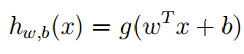z=ωTx+b $z = \omega^Tx+b$，那么：如果 z>=0 $z >= 0$ g(z)=1 $g(z) = 1$,如果 z<0 $z \lt 0$ g(z)=1 $g(z) = -1$ ω=[θ1,θ2...θn]T $\omega = [\theta_1,\theta_2...\theta_n]^T$ b=θ0 $b = \theta_0$ xRn $x \in \mathbb R^n$

hθ(x)=g(θTx)

# 函数间隔和几何间隔（Functional and geometric margins）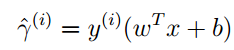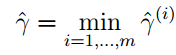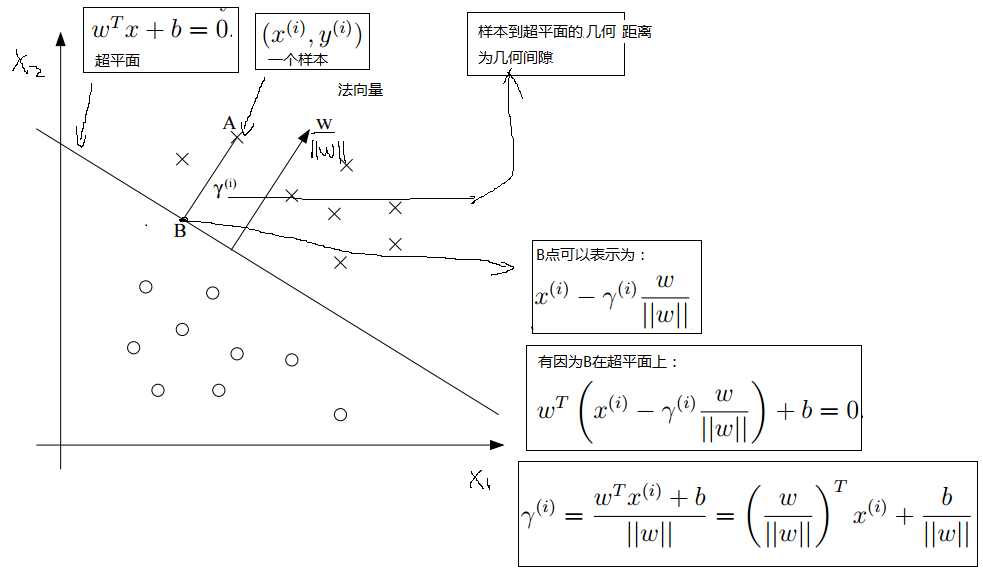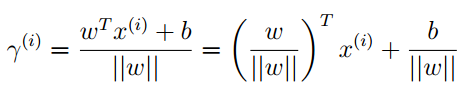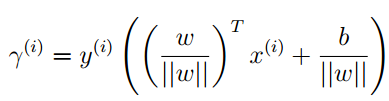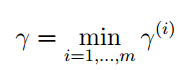# 最大间隔分类器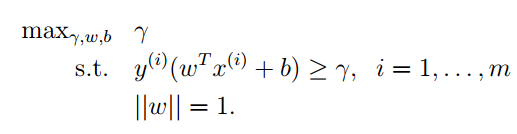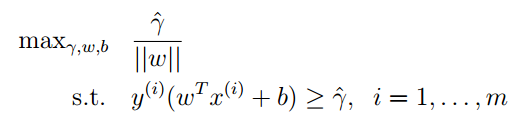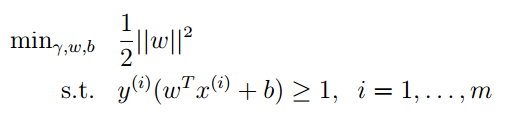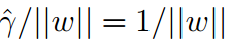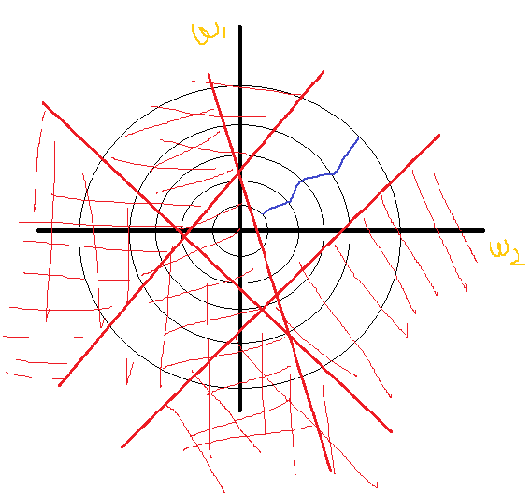# 一些与拉格朗日极值有关的理论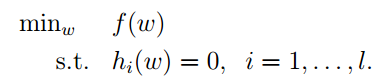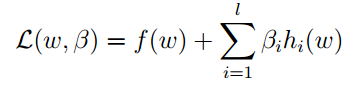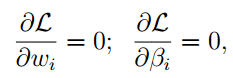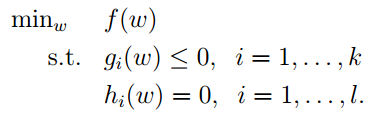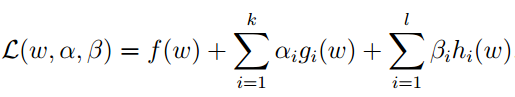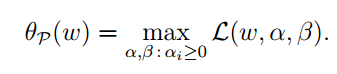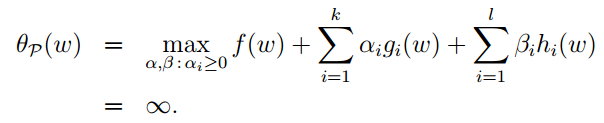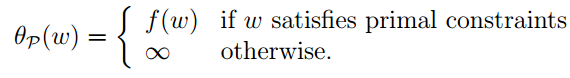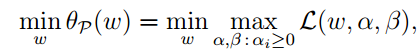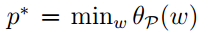p $p^*$是函数的最优值。下来我们在看一个稍微有点不同的问题，事实上是 θp(ω) $\theta_p(\omega)$的对偶问题，下标 ddual $d（dual）$表示对偶的意思：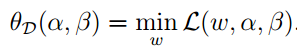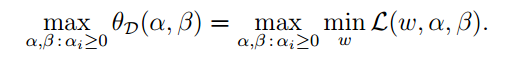1. 目标函数值相同时，各自的解为最优解
2. 若原问题有最优解，那么对偶问题也有最优解，且目标函数值相同
3. 还有互补松弛性，无界性，对称性等等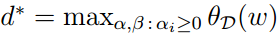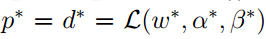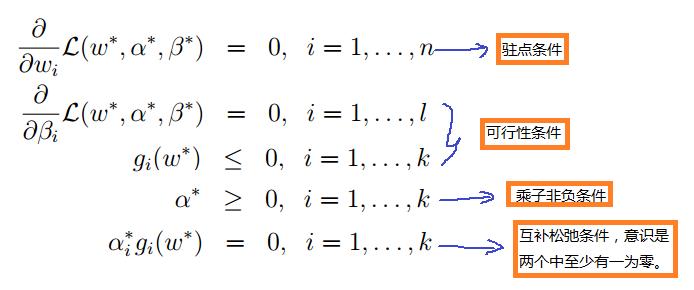# 最大间隔分类器的求解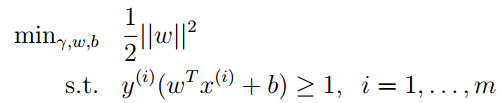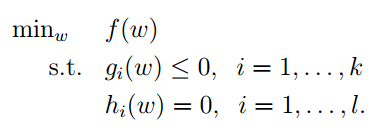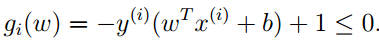Andrew Ng老师说在实验中，KKT条件的乘子非负条件一般都是成立的，即 αi>0 $\alpha_i > 0$，那么由互补松弛条件就可以得到 gi(ω)=0 $g_i(\omega) = 0$，即 y(i)(ωTx(i)+b)=1 $y^{(i)}(\omega^Tx^{(i)}+b) = 1$。在最大间隔分类器的约束条件中 ω $\omega$就是一个单位向量。所以说 y(i)(ωTx(i)+b) $y^{(i)}(\omega^Tx^{(i)}+b)$就是几何间隔。看下图：

31.png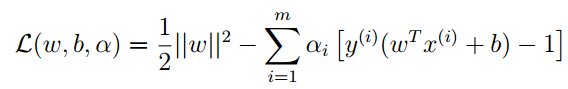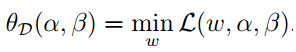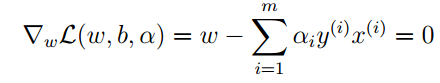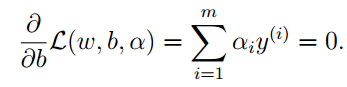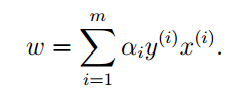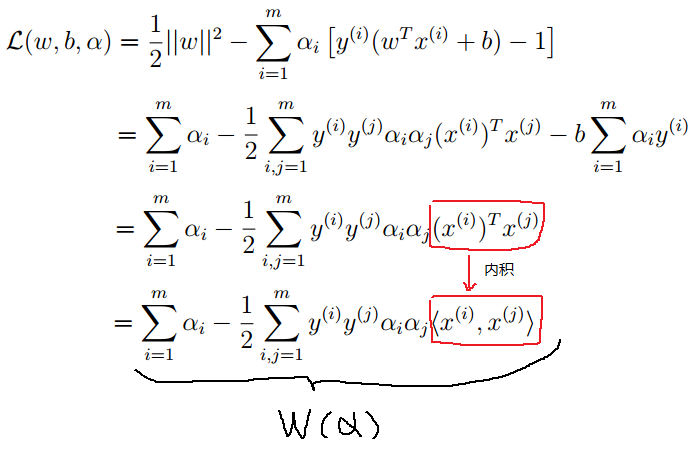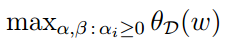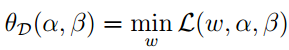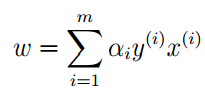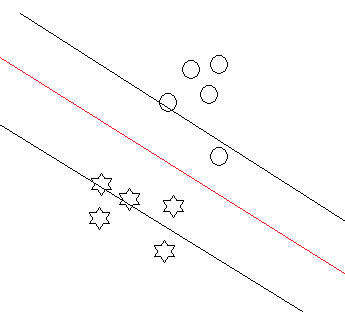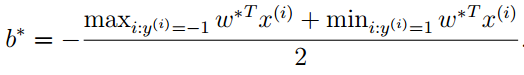# 总结

end
2015/12/31 15年的最后一天我还在学习，挺好。### .net core 3.1 swagger文档_chafferer_的博客-程序员秘密

.net core 3.1 swagger文档1.安装包Swashbuckle.AspNetCore2.在Startup.cs 配置swagger 在ConfigureServices中添加代码services.AddSwaggerGen(c =&gt; { c.SwaggerDoc("v1", new OpenApiInfo { Title = "My API", Version = "v1" }); ...

### dojo入门学习_chagaostu的博客-程序员秘密

<!DOCTYPE html PUBLIC "-//W3C//DTD HTML 4.01//EN" "http://www.w3.org/TR/html4/strict.dtd"> Dojo Toolkit Test Page <mce:script type="text/javascript" src="js/dojotoolki

### mysql 5.7.14-win32_MySQL mysql-5.7.14-win32 压缩包(免安装版) 配置步骤_weixin_39856630的博客-程序员秘密

==MySQL mysql-5.7.14-win32 压缩包(免安装版) 配置步骤==1. 自MySQL官网上下载zip版文件。文件名称为：mysql-5.7.14-win32.zip2. 解压缩zip文件，测试路径为 D:\Coding\mysql-5.7.14-win323. 将解压缩路径下的bin路径添加到环境变量中：-------------------------------------...

### postfix main.cf配置参数_choumu8867的博客-程序员秘密

main.cf文件内容的格式： 每个逻辑行是name=value的形式，=两边的空格和逻辑行最后面的空格都将忽略掉。 空行，以#开头的行或者全是空格的行都将忽略。 逻辑行不以空格开始，如果某一行以空格开始则表示逻辑行的连续。 某些参数可能与其他参数产生关联： ...

### gb2312 转 unicode 转 utf-8_admyww的博客-程序员秘密

#include #include int GBKConvertTableUnicode[] = {{0xb0a1, 0x554a},/*啊*/{0xb0a2, 0x963f},/*阿*/{0xb0a3, 0x57c3},/*埃*/{0xb0a4, 0x6328},/*挨*/{0xb0a5, 0x54ce},/*哎*/{0xb0a6, 0x5509},/*唉*/{0xb0a7, 0x54c0